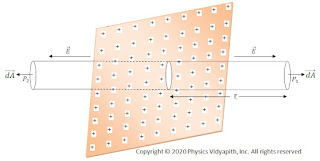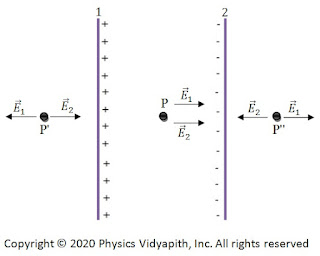### Electric field intensity due to uniformly charged plane sheet and parallel sheet

Electric field intensity due to a uniformly charged infinite plane thin sheet:

Let us consider, A plane charged sheet (It is a thin sheet so it will have surface charge distribution whether it is a conducting or nonconducting sheet) whose surface charge density is $\sigma$. From symmetry, Electric field intensity is perpendicular to the plane everywhere and the field intensity must have the same magnitude on both sides of the sheet. Let point $P_{1}$ and $P_{2}$ be the two-point on the opposite side of the sheet.

To use Gaussian law, we construct a cylindrical Gaussian surface of cross-section area $\overrightarrow{dA}$, which cuts the sheet, with points $P_{1}$ and $P_{2}$. The electric field $\overrightarrow{E}$ is normal to end faces and is away from the plane. Electric field $\overrightarrow{E}$ is parallel to cross-section area $\overrightarrow{dA}$. Therefore the curved cylindrical surface does not contribute to the flux i.e. $\oint \overrightarrow{E} \cdot \overrightarrow{dA}=0$.Hence the total flux is equal to the sum of the contribution from the two end faces. Thus, we get

$\phi_{E}=\int_{A} \overrightarrow{E} \cdot \overrightarrow{dA}+\int_{A} \overrightarrow{E} \cdot \overrightarrow{dA}$

$\phi_{E}= \int_{A} E \: dA \:cos 0^{\circ} +\int_{A} E \: dA \:cos 0^{\circ} \quad \left \{\because \theta=0^{\circ} \right \}$Infinite plane thin sheet

$\phi_{E}= \int_{A} E \: dA +\int_{A} E \: dA$

$\phi_{E}= \int_{A} 2E \: dA$

$\phi_{E}= 2E \int_{A} \: dA$

$\phi_{E}= 2E\:A$

$\frac{q}{\epsilon_{0}}=2E\:A \qquad \left \{\because \phi_{E}=\frac{q}{\epsilon_{0}} \right \}$

$E=\frac{q}{2\epsilon_{0} A}$

$\because q=\sigma A$, So the above equation can be written as:

$E=\frac{\sigma A}{2\epsilon_{0} A}$

$E=\frac{\sigma}{2\epsilon_{0}}$

Electric field intensity due to the uniformly charged infinite conducting plane thick sheet or Plate:

Let us consider that a large positively charged plane sheet having a finite thickness is placed in the vacuum or air. Since it is a conducting plate so the charge will be distributed uniformly on the surface of the plate. Let $\sigma$ be the surface charge density of the charge

Let's take a point $P$ close to the plate at which electric field intensity has to determine. Since there is no charge inside the conducting plate, this conducting plate can be assumed as equivalent to two plane sheets of charge i.e sheet 1 and sheet 2.Plane Charged Plate

The magnitude of the electric field intensity $\overrightarrow {E_{1}}$ at point $P$ due to sheet 1 is →

$E_{1}=\frac{\sigma}{2\epsilon_{0}}$    (away from sheet 1)

The magnitude of the electric field intensity $\overrightarrow {E_{2}}$ at point $P$ due to sheet 2 is →

$E_{2}=\frac{\sigma}{2\epsilon_{0}}$    (away from sheet 2)

Since $\overrightarrow {E_{1}}$ and $\overrightarrow {E_{2}}$ are in the same direction, the magnitude of resultant intensity $\overrightarrow {E}$ at point $P$ due to both the sheet is →

$E=E_{1}+E_{2}$

$\because \quad E=\frac{\sigma}{2\epsilon_{0}}+\frac{\sigma}{2\epsilon_{0}}$

$E=\frac{\sigma}{\epsilon_{0}}$

The resultant electric field will be away from the plate. If the plate is negatively charged, the electric field intensity $\overrightarrow {E}$ would be directed toward the plate.

We have obtained the above formula for a 'plane' charged conductor. In fact, it holds for the electric field intensity 'just' outside a charged conductor of any shape.

Electric field intensity due to two Infinite Parallel Charged Sheets:

When both sheets are positively charged:

Let us consider, Two infinite, plane, sheets of positive charge, 1 and 2 are placed parallel to each other in the vacuum or air. Let $\sigma_{1}$ and $\sigma_{2}$ be the surface charge densities of charge on sheet 1 and 2 respectively.Likely positive charged sheet
Let $\overrightarrow {E_{1}}$ and $\overrightarrow {E_{2}}$ be the electric field intensities at any point due to sheet 1 and sheet 2 respectively. Then,

The electric field intensity at points $P'$ →

$E_{1}=\frac{\sigma_{1}}{2\epsilon_{0}}$    (away from sheet 1)

$E_{2}=\frac{\sigma_{2}}{2\epsilon_{0}}$    (away from sheet 2)

Since, Electric field intensities $\overrightarrow {E_{1}}$ and $\overrightarrow {E_{2}}$ are in the same direction, the magnitude of resultant intensity at point $P'$ is given by →

$E=E_{1}+E_{2}$

$E=\frac{\sigma_{1}}{2 \epsilon_{0}}+\frac{\sigma_{2}}{2 \epsilon_{0}}$

$E=\frac{1}{2 \epsilon_{0}} \left (\sigma_{1}+\sigma_{2} \right )$

If both sheets have equal charge densities $\sigma$ i.e. $\sigma_{1}=\sigma_{2}=\sigma$, Then above equation can be written as:

$E=\frac{\sigma}{ \epsilon_{0}}$

This electric field intensity would be away from both sheet 1 and sheet 2.

The electric field intensity at points $P$→

Electric field intensity at point $P$ due to sheet 1 is →

$E_{1}=\frac{\sigma_{1}}{2\epsilon_{0}}$    (away from sheet 1)

$E_{2}=\frac{\sigma_{2}}{2\epsilon_{0}}$    (away from sheet 2)

Now, both electric field intensities $\overrightarrow{E_{1}}$ and $\overrightarrow{E_{2}}$ are in opposite direction. The magnitude of resultant electric field $\overrightarrow{E}$ at point $P$ is given by

$E= E_{1}-E_{2}$

$E=\frac{\sigma_{1}}{2 \epsilon_{0}}-\frac{\sigma_{2}}{2 \epsilon_{0}}$

$E=\frac{1}{2 \epsilon_{0}} \left (\sigma_{1}-\sigma_{2} \right )$

If both sheets have equal charge densities $\sigma$ i.e. $\sigma_{1}=\sigma_{2}=\sigma$, Then above equation can be written as:

$E=0$

The electric field intensity at points $P''$ → $E_{1}=\frac{\sigma_{1}}{2\epsilon_{0}}$    (away from sheet 1)

$E_{2}=\frac{\sigma_{2}}{2\epsilon_{0}}$    (away sheet 2)

Since, Electric field intensities $\overrightarrow {E_{1}}$ and $\overrightarrow {E_{2}}$ are in the same direction, the magnitude of resultant intensity at point $P''$ is given by →

$E=E_{1}+E_{2}$

$E=\frac{\sigma_{1}}{2 \epsilon_{0}}+\frac{\sigma_{2}}{2 \epsilon_{0}}$

$E=\frac{1}{2 \epsilon_{0}} \left (\sigma_{1}+\sigma_{2} \right )$

If both sheets have equal charge densities $\sigma$ i.e. $\sigma_{1}=\sigma_{2}=\sigma$, Then above equation can be written as:

$E=\frac{\sigma}{ \epsilon_{0}}$

This electric field intensity would be away from both sheet 1 and sheet 2.

When one-sheet is positively charged and the other sheet negatively charged:

Let us consider two sheets 1 and 2 of positive and negative charge densities $\sigma_{1}$ and $\sigma_{2}$ ($\sigma_{1} > \sigma_{2}$)Unlike charged parallel Sheet
The electric field intensities at point $P'$ →

$E_{1}=\frac{\sigma_{1}}{2\epsilon_{0}}$    (away from sheet 1)

$E_{2}=\frac{\sigma_{2}}{2\epsilon_{0}}$    (toward from sheet 2)

The magnitude of the resultant electric field $E$

$E=E_{1}-E_{2}$

$E= \frac{1}{2\epsilon_{0}} \left ( \sigma_{1}- \sigma_{2}\right )$

If both sheets have equal charge densities $\sigma$ i.e. $\sigma_{1}=\sigma_{2}=\sigma$, Then above equation can be written as:

$E=0$

The electric field intensities at point $P$ →

$E_{1}=\frac{\sigma_{1}}{2\epsilon_{0}}$    (away from sheet 1)

$E_{2}=\frac{\sigma_{2}}{2\epsilon_{0}}$    (towards sheet 2)

Since, Electric field intensities $\overrightarrow {E_{1}}$ and $\overrightarrow {E_{2}}$ are in the same direction, the magnitude of resultant intensity at point $P$ is given by →

$E=E_{1}+E_{2}$

$E=\frac{\sigma_{1}}{2 \epsilon_{0}}+\frac{\sigma_{2}}{2 \epsilon_{0}}$

$E=\frac{1}{2 \epsilon_{0}} \left (\sigma_{1}+\sigma_{2} \right )$

If both sheets have equal charge densities $\sigma$ i.e. $\sigma_{1}=\sigma_{2}=\sigma$, Then above equation can be written as:

$E=\frac{\sigma}{ \epsilon_{0}}$

The electric field intensities at point $P''$ →

$E_{1}=\frac{\sigma_{1}}{2\epsilon_{0}}$    (away from sheet 1)

$E_{2}=\frac{\sigma_{2}}{2\epsilon_{0}}$    (toward from sheet 2)

The magnitude of the resultant electric field $E$ →

$E=E_{1}-E_{2}$

$E= \frac{1}{2\epsilon_{0}} \left ( \sigma_{1}- \sigma_{2}\right )$

If both sheets have equal charge densities $\sigma$ i.e. $\sigma_{1}=\sigma_{2}=\sigma$, Then above equation can be written as:

$E=0$

From the above expression, we can conclude that the magnitude of $E$ is free from the 'position' of the point taken in the electric field between the sheet and outside the sheet. It is also shown that the electric field between the sheet is uniform everywhere and independent of separation between the sheets.